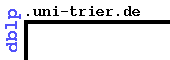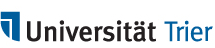# 4. IFIP Congress 1968, Volume 1: Edinburgh, UK

A. J. H. Morrell (Ed.): Information Processing 68, Proceedings of IFIP Congress 1968, Edinburgh, UK, 5-10 August 1968, Volume 1 - Mathematics, Software.

## Part 1 - Mathematics

### Partial Differential Equations

• Paul Concus: Numerical solution of the minimal surface equation by block nonlinear successive overrelaxation. 153-158 BibTeX
• J. F. Croce-Spinelli: A method for identification problems in partial differential equations. 159-163 BibTeX
• J. C. Mason: Chebyshev methods for separable partial differential equations. 164-169 BibTeX
• Robert Vichnevetsky: Application of hybrid computers to the integration of partial differential equations of the first and second order. 170-178 BibTeX
• M. Zlámal: On mildly nonlinear elliptic boundary value problems. 179-182 BibTeX

### Ordinary Differential Equations

• Germund Dahlquist: A numerical method for some ordinary differential equations with large Lipschitz constants. 183-186 BibTeX
• C. William Gear: The automatic integration of stiff ordinary differential equations. 187-193 BibTeX
• Fred T. Krogh: A variable-step, variable-order multistep method for the numerical solution of ordinary differential equations. 194-199 BibTeX
• Michael R. Osborne: A new method for the integration of stiff systems of ordinary differential equations. 200-204 BibTeX